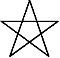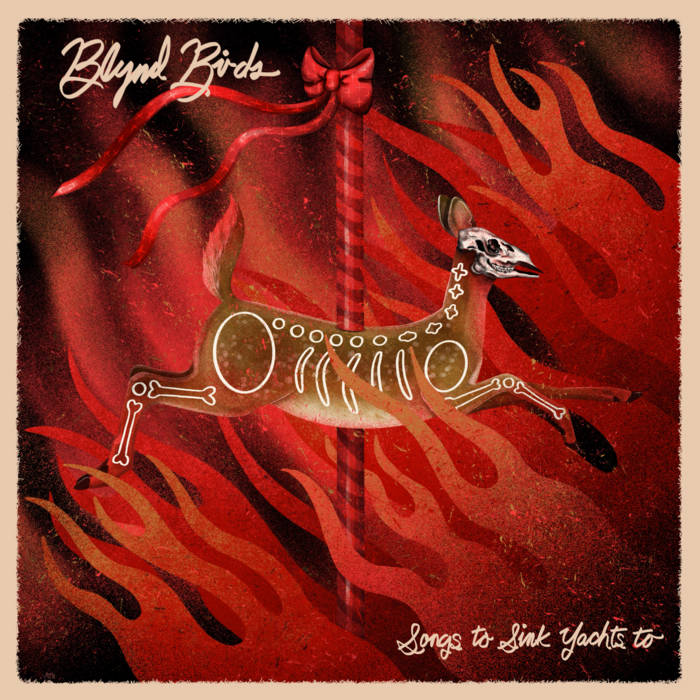Art, History, Music, Politics, and Spirituality For The Modern Alchemist – circulation in over 129 countries

# Sign of Amaterasu Ohkami Begins Year 18,004 Begins March 19th 2016

There are eight months in the Calendar of Mu, sometimes referred to as the Nyarzirian Calendar. Each month is consists of forty-five days, and represents a system of the eight hexagrams moving through the five elements. The Ninzuwu Priest/Priestess invokes the mudra/mantra formulae associated with each hexagram that rules a particular day after invoking the Armor of Amaterasu Ohkami. The chart below illustrates the daily Vasuh letters invoked and the ruling “deity” for the said hexagram:

1. March 19th, 2016 = Hahun = 14th Hexagram = Zhee-Bnhu-Aum
2. March 20th, 2016 = Shapash = 21st Hexagram =Phe-Aum-Nzu
3. March 21st 2016 = Amaterasu = 30th Hexagram = Zhee-Lewhu
4. March 22nd 2016 = Nuru = 35th Hexagram = Bnhu
5. March 23rd 2016 = Yu Nin = 38th Hexagram = Phe-Aum-Shki
6. March 24th 2016 = Kagutsuchi = 50th Hexagram = Hmu-Hmu
7. March 25th 2016 = Fujiyama = 56th Hexagram = Bnhu-Phe
8. March 26th 2016 = Tengu = 64th Hexagram = Zhee-Tuu-Zhee-Hmu
9. March 27th 2016 =Entering Earth Element
10. March 28th 2016 = Hahun = 14th Hexagram = Zhee-Bnhu-Aum
11. March 29th 2016 = Shapash = 21st Hexagram =Phe-Aum-Nzu
12. March 30th 2016 = Amaterasu = 30th Hexagram = Zhee-Lewhu
13. March 31st 2016 = Nuru = 35th Hexagram = Bnhu
14. April 1st 2016 = Yu Nin = 38th Hexagram = Phe-Aum-Shki
15. April 2nd 2016 = Kagutsuchi = 50th Hexagram = Hmu-Hmu
16. April 3rd 2016 = Fujiyama = 56th Hexagram = Bnhu-Phe
17. April 4th 2016 = Tengu = 64th Hexagram = Zhee-Tuu-Zhee-Hmu
18. April 5th 2016 =Entering Water Element
19. April 6th 2016 = Hahun = 14th Hexagram = Zhee-Bnhu-Aum
20. April 7th 2016 = Shapash = 21st Hexagram =Phe-Aum-Nzu
21. April 8th 2016 = Amaterasu = 30th Hexagram = Zhee-Lewhu
22. April 9th 2016 = Nuru = 35th Hexagram = Bnhu
23. April 10th 2016 = Yu Nin = 38th Hexagram = Phe-Aum-Shki
24. April 11th 2016 = Kagutsuchi = 50th Hexagram = Hmu-Hmu
25. April 12th 2016 = Fujiyama = 56th Hexagram = Bnhu-Phe
26. April 13th 2016 = Tengu = 64th Hexagram = Zhee-Tuu-Zhee-Hmu
27. April 14th 2016 =Entering Fire Element
28. April 15th 2016 = Hahun = 14th Hexagram = Zhee-Bnhu-Aum
29. April 16th 2016 = Shapash = 21st Hexagram =Phe-Aum-Nzu
30. April 17th 2016 = Amaterasu = 30th Hexagram = Zhee-Lewhu
31. April 18th 2016 = Nuru = 35th Hexagram = Bnhu
32. April 19th 2016 = Yu Nin = 38th Hexagram = Phe-Aum-Shki
33. April 20th 2016 = Kagutsuchi = 50th Hexagram = Hmu-Hmu
34. April 21st 2016 = Fujiyama = 56th Hexagram = Bnhu-Phe
35. April 22nd 2016 = Tengu = 64th Hexagram = Zhee-Tuu-Zhee-Hmu
36. April 23rd 2016 =Entering Air Element
37. April 24th 2016 = Hahun = 14th Hexagram = Zhee-Bnhu-Aum
38. April 25th 2016 = Shapash = 21st Hexagram =Phe-Aum-Nzu
39. April 26th 2016 = Amaterasu = 30th Hexagram = Zhee-Lewhu
40. April 27th 2016 = Nuru = 35th Hexagram = Bnhu
41. April 28th 2016 = Yu Nin = 38th Hexagram = Phe-Aum-Shki
42. April 29th 2016 = Kagutsuchi = 50th Hexagram = Hmu-Hmu
43. April 30th 2016 = Fujiyama = 56th Hexagram = Bnhu-Phe
44. May 1st 2016 = Tengu = 64th Hexagram = Zhee-Tuu-Zhee-Hmu
45. May 2nd 2016 =Entering Void Element

#### You may have missed#### Covered in Blood -Joshua Ketchmark#### Joshua Ketchmark’s New Album Is A Masterpiece: Blood